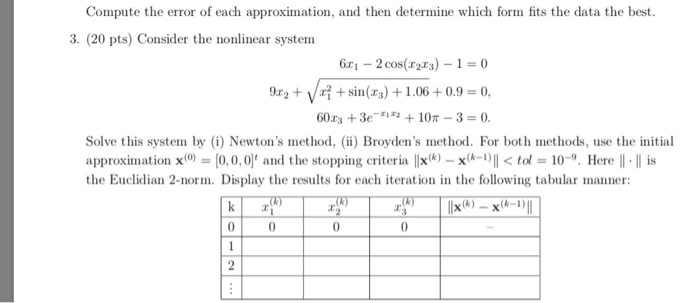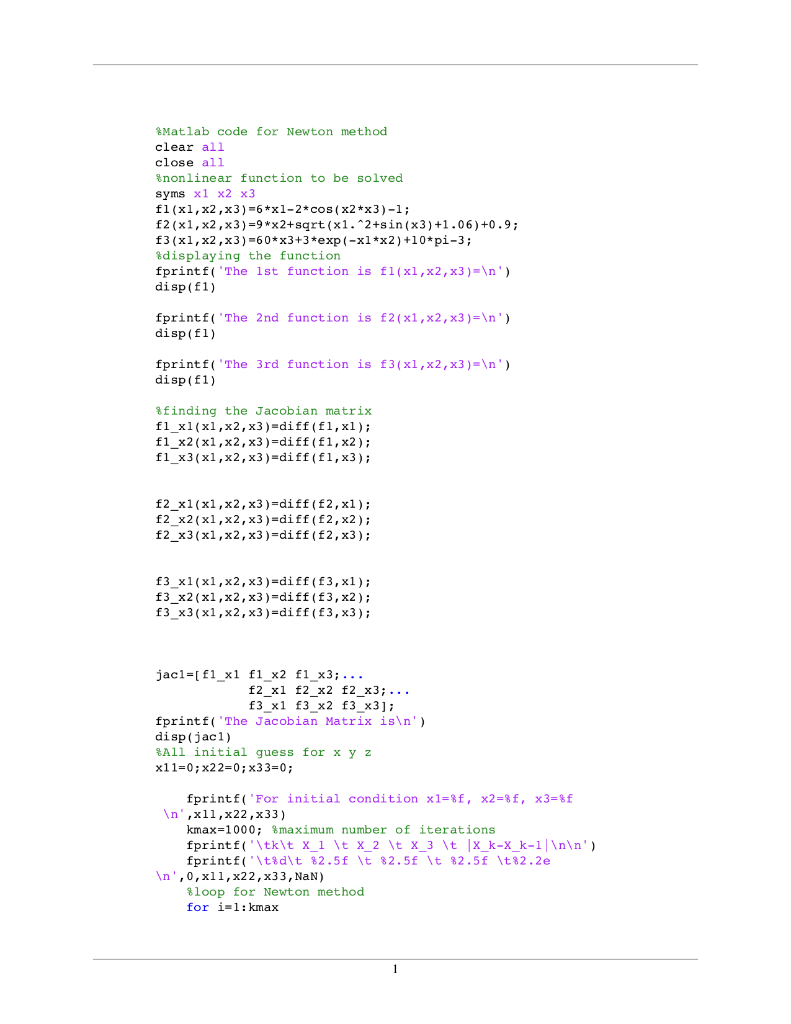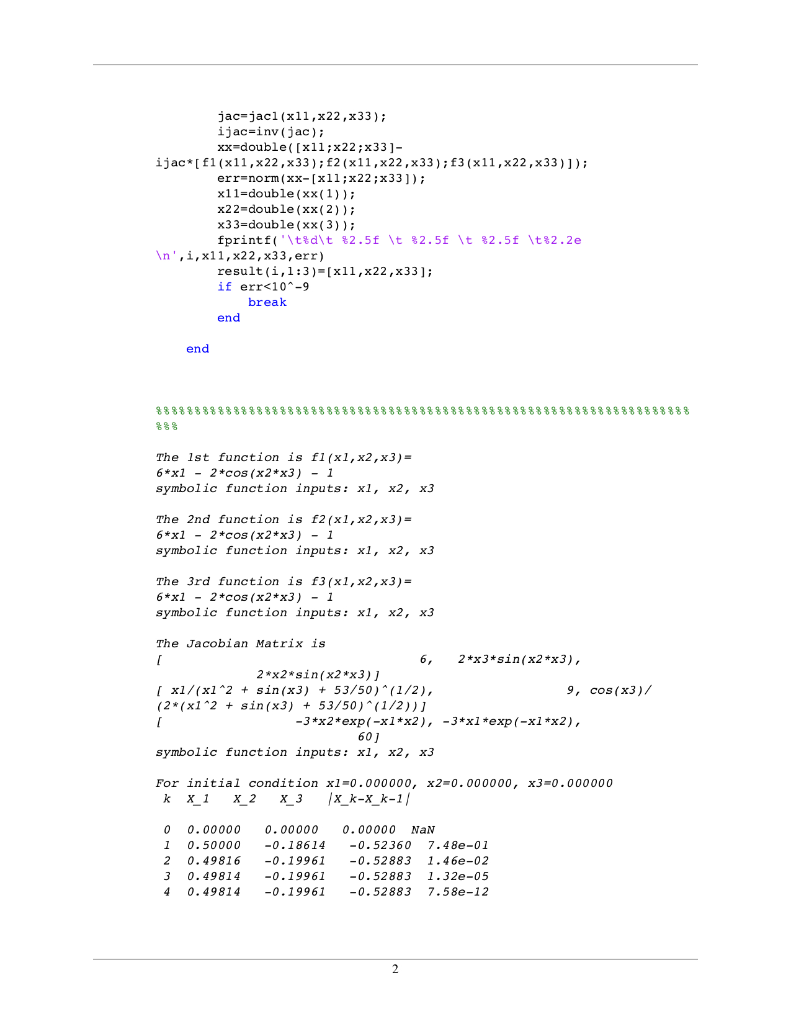Homework Help Question & Answers

Compute the error of each approximation, and then determine which form fits the data the best 3. ...Compute the error of each approximation, and then determine which form fits the data the best 3. (20 pts) Consider the nonlinear system 6x1 2 cos(3)-1-0 9r2 + Vr| + sin(za) + 1.06 + 0.9 = 0, Solve this system by i) Newton's method, (ii) Broyden's method. For both methods, use the initial approximation x() = [0,0,0], and the stopping criteria llx(k) X(k 1) I%Matlab code for Newton method
clear all
close all
%nonlinear function to be solved
syms x1 x2 x3
f1(x1,x2,x3)=6*x1-2*cos(x2*x3)-1;
f2(x1,x2,x3)=9*x2+sqrt(x1.^2+sin(x3)+1.06)+0.9;
f3(x1,x2,x3)=60*x3+3*exp(-x1*x2)+10*pi-3;
%displaying the function
fprintf('The 1st function is f1(x1,x2,x3)=\n')
disp(f1)

fprintf('The 2nd function is f2(x1,x2,x3)=\n')
disp(f1)

fprintf('The 3rd function is f3(x1,x2,x3)=\n')
disp(f1)

%finding the Jacobian matrix
f1_x1(x1,x2,x3)=diff(f1,x1);
f1_x2(x1,x2,x3)=diff(f1,x2);
f1_x3(x1,x2,x3)=diff(f1,x3);

f2_x1(x1,x2,x3)=diff(f2,x1);
f2_x2(x1,x2,x3)=diff(f2,x2);
f2_x3(x1,x2,x3)=diff(f2,x3);

f3_x1(x1,x2,x3)=diff(f3,x1);
f3_x2(x1,x2,x3)=diff(f3,x2);
f3_x3(x1,x2,x3)=diff(f3,x3);

jac1=[f1_x1 f1_x2 f1_x3;...
f2_x1 f2_x2 f2_x3;...
f3_x1 f3_x2 f3_x3];
fprintf('The Jacobian Matrix is\n')
disp(jac1)
%All initial guess for x y z
x11=0;x22=0;x33=0;

fprintf('For initial condition x1=%f, x2=%f, x3=%f \n',x11,x22,x33)
kmax=1000; %maximum number of iterations
fprintf('\tk\t X_1 \t X_2 \t X_3 \t |X_k-X_k-1|\n\n')
fprintf('\t%d\t %2.5f \t %2.5f \t %2.5f \t%2.2e\n',0,x11,x22,x33,NaN)
%loop for Newton method
for i=1:kmax

jac=jac1(x11,x22,x33);
ijac=inv(jac);
xx=double([x11;x22;x33]-ijac*[f1(x11,x22,x33);f2(x11,x22,x33);f3(x11,x22,x33)]);
err=norm(xx-[x11;x22;x33]);
x11=double(xx(1));
x22=double(xx(2));
x33=double(xx(3));
fprintf('\t%d\t %2.5f \t %2.5f \t %2.5f \t%2.2e\n',i,x11,x22,x33,err)
result(i,1:3)=[x11,x22,x33];
if err<10^-9
break
end

end

%%%%%%%%%%%%%%%%%%%%%%%%%%%%%%%%%%%%%%%%%%%%%%%%%%%%%%%%%%%%%%%%%%%%%%%%

Add Answer of: Compute the error of each approximation, and then determine which form fits the data the best 3. ...
More Homework Help Questions Additional questions in this topic.

• Assessing Random Error, Confounding, and Effect Modification Although researchers do their best to reduce error within every study, there will always be error

Need Online Homework Help?

Get FREE EXPERT Answers
WITHIN MINUTES
Related Questions